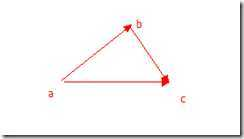數學趣味----抽象

1.問題：將下面每一組4個數經過加減乘除得到24.（是不是看上去很簡單哦）

1 .第一反應就是5*5-1 = 24，可是少用一個5;

2.那么我們換個方向把左右2邊恒等不變，但是又多一個5來呢？

3. 左右同時除5：

(2)   (3*7+3)/7 = 24/7 ---> (3+3/7)*7 = 24

(3)   (4*7-4)/7 = 24/7----> (4-4/7)*7 = 24

2.求證 (a-b)2 = a2 – 2ab + b2

(a - b)2
= (a - b)(a - b) %%定義
= a(a - b) - b(a - b) %%分配律
= (aa - ab) +(- ba + bb)%%分配律
= a2 - ab - ab +b2 %%乘法交換律
= a2 -2ab + b2 %%合并律ab2 = ca2 +cb2 – 2*ca*cb*cosc

1. 把橢圓先拉成一個圓橫坐標不變，縱坐標變成和橫坐標相等，(x,y) ---->(x,a/b*y).  【注意這里的x，y不是指半徑】。

2. 橢圓---->圓(方程）  x2/a2 + y2/b2 = 1   ----> 左右乘a2 ---->x2+(a2/b2)*y2 =a2 ------>所以只要y變成原來的a/b倍，那么就是一個半徑為a的圓啦

3. S 擴大為圓的面積 Pi*a2 = (a/b)*S  【拉伸了a/b倍】

4. S = (Pi*a2)(b/a) = Pi*a

(1)
(1)? 2014 mamicode.com 版權所有 京ICP備13008772號-2